# Floating Point Numbers

SparkiDuino
Easy

### Lessons You Should Know

Floating point numbers are numbers that have decimal points. Unlike integers, floating point numbers let you use partial numbers. This means they are good to use when you do not know if there will be numbers after a decimal point, like when you divide and keep the remainder. Since “floating point numbers” is a mouthful to use every time programmers want to refer to these types of numbers this type of variable is usually called a “float.” Floats can also can be much bigger than integers. As big as 2^128 or small as 2^-127. That’s very, very big, for example: 340,282,340,000,000,000,000,000,000,000,000,000,000.0 or very, very small: 0.000000000000000000000000000000000000000000000014012985 Here’s what we’ll be covering on this page:

#### Declaring a Float Variable

You create a float variable by declaring it in the same way that you declare an integer, except instead of using int you use float: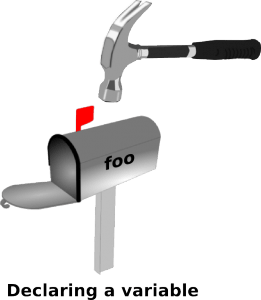This will create a float named “foo” with a stored value of 0.0.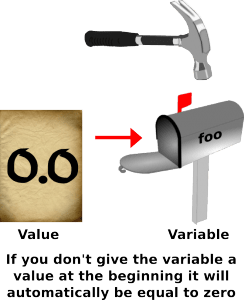#### Assigning Values (Putting Information in the Float Variables)

You can then change the float value by assigning a value to the variable. (Just like you did with integers.) This is done simple by writing the variable name followed by an equals sign, followed by the value you want to put in the variable. Then you end your command for assigning a variable with the all important semicolon. Everything else is pretty self explanatory but the semicolon is there so the computer knows that it has reached the end of a line of instructions that should now be executed. You can think of the semicolon as a green light that tells the computer it has all the parts of a command and it can start moving ones and zeros around. Here’s what it looks like when we assign the value of eight point zero zero six to the float variable “foo.”You can think of a float variable like a mailbox with a letter in it. You can write any number on the paper inside the letter and the robot will look inside the letter anytime it sees the variable name that is on the mailbox. Then the robot will just insert whatever number is on the letter into the code it is running in place of the variable. You can also declare a float and assign a number to the variable in the same line of code. Here’s what it looks like to declare a float variable named “foo ” with the initial value of six point seven three: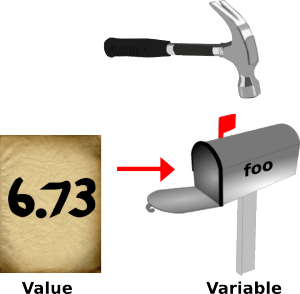#### Doing Math with Floats

Now that you know about floats, try using them instead of integers with the code we covered before: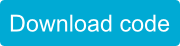Before, when we divided 10 by 4 using an integer we got an answer of 2. You will see that foo/blerg now gives the correct result since we used a float!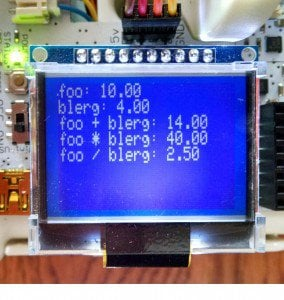#### Float Variables Aren’t Always Exact

Floats are useful when you need to store partial numbers, very big numbers, or very small numbers. The downside is that unlike integers they can only store so much of the number. They are also not 100% accurate. If you add 1+1 for an integer, you get 2. If you add 1+1 using a float, you get: 2.00000000000001. This is called a rounding error. Most of the time, this is okay, but 2 does not equal 2.00000000000001, so it is not useful when you need to exactly compare numbers. To avoid problems happening in your code, only use floats when you cannot use integers.

or

## Next Step:

You will often need to work with more than numbers. Sometimes you need to write text. For that, you will need to learn about characters: